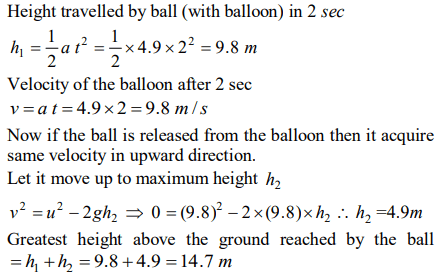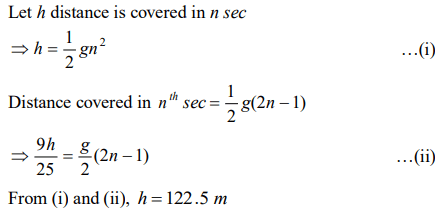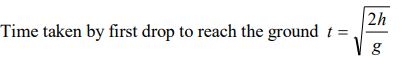## Motion in a Straight Line Questions and Answers Part-15

1. A body thrown with an initial speed of 96 ft / sec reaches the ground after $\left(g=32 ft \diagup sec^{2}\right)$
a) 3 sec
b) 6 sec
c) 12 sec
d) 8 sec

Explanation:2. A stone is dropped from a certain height which can reach the ground in 5 second. If the stone is stopped after 3 second of its fall and then allowed to fall again, then the time taken by the stone to reach the ground for the remaining distance is
a) 2 sec
b) 3 sec
c) 4 sec
d) None of these

Explanation:3. A man in a balloon rising vertically with an acceleration of $g=4.9 m \diagup sec^{2}$   releases a ball 2 sec after the balloon is let go from the ground. The greatest height above the ground reached by the ball is $\left(g=9.8 m \diagup sec^{2}\right)$
a) 14.7 m
b) 19.6 m
c) 9.8 m
d) 24.5 m

Explanation:4. A particle is dropped under gravity from rest from a height $h\left(g=9.8 m \diagup sec^{2}\right)$     and it travels a distance $9h\diagup25$   in the last second, the height h is
a) 100 m
b) 122.5 m
c) 145 m
d) 167.5 m

Explanation:5. A balloon is at a height of 81 m and is ascending upwards with a velocity of 12 m/s. A body of 2kg weight is dropped from it. If $g=10 m \diagup s^{2}$  , the body will reach the surface of the earth in
a) 1.5 s
b) 4.025 s
c) 5.4 s
d) 6.75 s

Explanation:6. An aeroplane is moving with a velocity u . It drops packet from a height h . The time t taken by the packet in reaching the ground will be
a) $\sqrt{\left(\frac{2g}{h}\right)}$
b) $\sqrt{\left(\frac{2u}{g}\right)}$
c) $\sqrt{\left(\frac{h}{2g}\right)}$
d) $\sqrt{\left(\frac{2h}{g}\right)}$

Explanation:7. Water drops fall at regular intervals from a tap which is 5 m above the ground. The third drop is leaving the tap at the instant the first drop touches the ground. How far above the ground is the second drop at that instant
a) 2.50 m
b) 3.75 m
c) 4.00 m
d) 1.25 m

Explanation:8. A ball is thrown vertically upwards from the top of a tower at $4.9 ms^{-1}$ . It strikes the pond near the base of the tower after 3 seconds. The height of the tower is
a) 73.5 m
b) 44.1 m
c) 29.4 m
d) None of these

Explanation:9. A rocket is fired upward from the earth's surface such that it creates an acceleration of $19.6 m \diagup sec^{2}$  . If after 5 sec its engine is switched off, the maximum height of the rocket from earth's surface would be
a) 245 m
b) 490 m
c) 980 m
d)735 m10. A bullet is fired with a speed of 1000 m / sec in order to hit a target 100 m away. If $g=10 m \diagup s^{2}$ , the gun should be aimed